New-Age Five Questions >> Dive Below the Surface of Process Functioning with Apparency Questions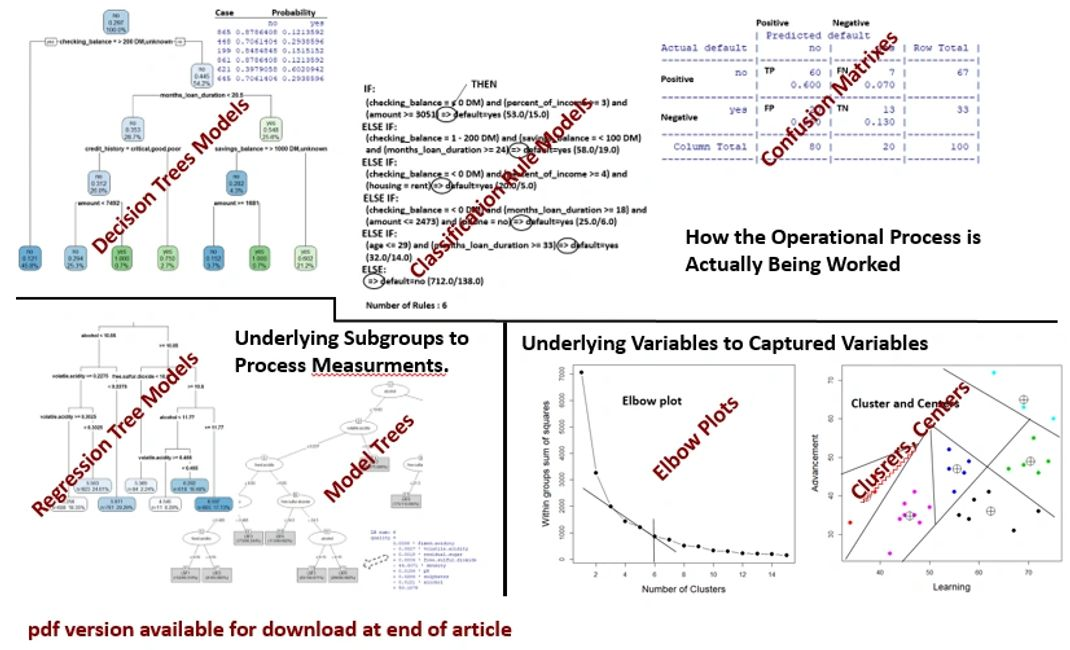By Richard G. Lamb, PE, CPA; Analytics4Strategy.com

What if we could dive below the surface of our operational processes? We would be able to ask questions of their functioning that are otherwise hidden to us—making the unapparent, apparent.

This article is a Plain-English explanation of “apparency” questioning. Apparency questioning is one of five core types of questioning—relationship, difference, time series, duration and apparency—all which play together to augment a team’s ability to reach for operational excellence.

However, the explanation we all want in the end is how to conduct the explained exploration. The article, “DMAIC Done the New-Age Way,” explains how the apparency questioning of this article, along with the four other core types of questioning are woven into the stages to define, measure, analyze, improve and control processes. Although presented in the context of DMAIC, the explanation is universal to any problem solving sequence.

The Nature of Apparency Questioning

The nature of apparency questioning is to explore operational processes below their surface. This is accomplished by reaching past the variables that the processes automatically capture in their computer systems. We mine the captured massive data to achieve three types of transparency—how the process is actually being worked, underlying subgroups to process measurements and underlying variables to captured variables.

How the Process is Being Worked: Any operational process is essentially a system of rules leading to outcome classification variables. If a process is conducted as it is charted or presumed to function, the aspect variables along the way will almost always arrive at the same classifications.

Rule analytics determine what rules are actually in effect and the degree the process is complying with the charted rules. Going the other way, the rules determined by the analytic can be used to classify outcomes which, for some reason, a classification was not recorded or needs to be known proactively. Rules can also be used to evaluate process stability by comparing rule compliance across periods in time.

Underlying Subgroups to Process Measurements: Measured outcomes are numeric variables such as dollars, hours, productivity, etc. Very often there are hidden homogenous subgroups to a measurement variable. In other words, they are not apparent in just the permutations of the variables captured in the database.

Once more, rule analytics come into play. However, now the rules are generated as subsets of a measured outcome. An example are the rules for natural groupings of average cost per case and the proportion the subgrouped cases are of the total cases. An example of capitalizing on such apparency is to determine the headcounts needed to conduct a process’s frontline actions.

Underlying Variables to Captured Variables: The previous first two types of apparency were formed with the specified target variables we select from the organization’s database. Now we are asking models to surprise us with a new target variable. We are surprised because the variable does not actually exist in the database, but is hidden beneath the interplay of variables.

Asking to be surprised is regarded as “unsupervised” analytics. In contrast, the previous two types of apparency are regarded as “supervised” because we gave them target variables. Consequently, it falls to the operatives, managers and SMEs to give the newly apparent variables their meaning; naming them as levels to a categorical variable (e.g., colors: red, white, blue).

Once revealed, each variable is available to be made a new variable to each case in the datasets we built from the system’s captured data. The resulting dataset becomes enhanced feedstock to almost every type of analytic.

What apparency questioning looks like and how to construct and interpret them will be explained—in plain English—by the next three sections.

How the Process is Being Worked

We begin with the overarching apparency question. How is the process actually being worked? This is explored by bringing to the surface the rules associated with the classifications of target outcome variables along the process. The question is answered with “decision tree models” and “classification rule models.”

Decision tree models are used to tease out the rules leading to two or more classifications. Classification rule models do the same, but generate an alternatively structured set of rules.

Decision Tree Models. Figure 1 shows a decision tree. To make the details presentable within the confines of an article, the decision tree has been arbitrarily truncated to four levels. Consequently, the probability of misclassification at the bottom of the figure are unnaturally high.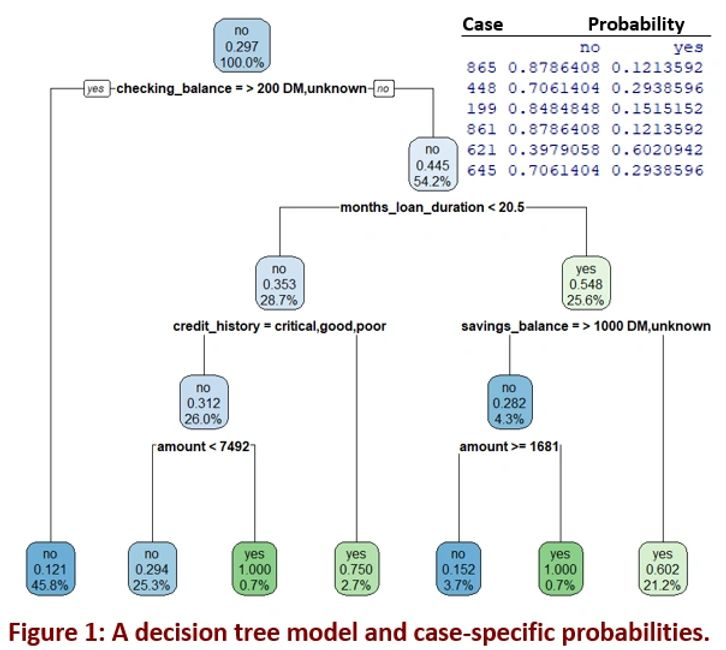The business purpose of the model is to improve a bank’s ability to grant loans to applicants who will not default. Ultimately, the findings will be tested from two directions. First, how frequently will the rules indicate a good loan that actually goes bad? Second, how frequently will good applicants be denied? Of course, both cases are a business loss to the bank.

The model learns to set its branches with respect to the categories or the numeric measurement splits of the indicative variables in the dataset—left if it meets the criteria (yes) of the level, right if it does not (no). At each level, the model decides which of the feed variables are most important after determining and comparing the most insightful split for each.

The model stops making splits when its innards determine that it has arrived at a level at which the model are no longer significantly improving its accuracy. Consequently, the method only pulls in the truly important indicator variables we gave it with which to learn the rules and make them apparent to us.

The end points for each branch are called a “leaf.” The southwest-most leaf of the tree contains 45.8 percent of the observations. It misclassified 12 percent of the observations and correctly classified the remaining 88 percent. It comes to the conclusion because the training dataset includes a specified target variable (default) of the actually defaulted and paid loans.

Notice that each trail along the branch is actually a rule leading to the classification at the leaf. Consequently, the improvement team can see how the process actually works to reach an outcome; as compared to how the process was designed to work.

The full model of Figure 1 would have found the optimum to be 10 levels. It would have revealed 17 leaves and associated rules. The tree would have also found that 10 of the 16 indicative variables are actually important to the model and in the order they appear top-to-bottom.

We started with the dataset of variables available to us because they are automatically captured in the firm’s operating systems. Now we can join two types of newly apparent data to the captured data. This creates a dataset upon which to strengthen the team’s ability to explore and control the process with the other analytics of questioning—relationship, difference, time series, and duration.

First, we can construct the rules of the tree to be a variable. Second, the distinctive probabilities for each case (see the inset to Figure 1) can be made a variable to the standard data.

Let’s make another distinction with respect to the purpose of our questioning. We may be only interested in assessing a subject operation. This is compared to using a trained model to determine classifications for sets of data that have not yet been classified for some reason, data we wish to classify proactively and confirm process stability. By stability, we are comparing periods for significant change in accuracy as indicative of changing compliance and behavior.

The model shown in Figure 1 was actually built on such a scenario. Consequently, the trained model is built upon a large part of the source dataset. It is then tested with a holdout set from the same source dataset. Otherwise, we would have built the model on the full data set because our interest is limited to what actually happened in a given period.

The model of Figure 1 was formed upon 90 percent of the total dataset. Before we can use the model we must evaluate it as a “classifier.” To do so, we pour the holdout 10 percent of the dataset into the model. Thence, we evaluate the correct and incorrect classifications predicted by the model with respect to the test data.

Figure 2 shows the primary evaluation tool for classification models, called the “confusion matrix.” Before explaining the matrix, we must establish what is meant by “positive” and “negative.” The distinction as positive or negative is not a judgment of good or bad. It is only an analytical reference point.  In this case we have set our “positive” to be “no” default.If we get it right, we have a true positive (TP) or true negative (TN). If not, we have a false positive (FP) or false negative (FN). Each designation is shown in the matrix.

Let’s translate this essential nomenclature to plain English. From the matrix we are interested in an overarching question. If we were using the model to take, or not take an action, how often would we have made the correct decision? We can answer the question from three vantages.

First, for those cases that we took an action, how frequently did we get it right? Overall, the model got it right 83 percent of the time ((20+7)/100). Second, whenever the bank decided to grant the loan, it would have been the correct decision 89.6 percent (60/67) of the time. Third, whenever the bank decided to not grant the loan, it would have been the correct decision only 39.3 percent (13/33) of the time.

Accordingly, for the operational process leading to granting or rejecting loans we would consider the consequences of either choice to the bank. It follows that we would try to improve the model. As part of that, we may insert the relative costs of bad choices in the model so that it can learn to adjust its branches, variables and splits to reflect the cost.

Further yet, we may try methods that often give us stronger tree models. They are called bootstrapping, boosting and random forests. These are outside the scope of this article, but arrive at the same type of conclusions. We would run each type, compare their confusion matrixes and other measures, and select the best for our final purpose.

Classification Rule Models. There is another method to seek out rules back from classifications. It is the classification rules model. The method presents what is learned in the form of a logical “if this happens, then that happens or else” (if-then-else) statements. Of course, IF-THEN-ELSE is inherent to decision trees. However, in contrast to the top-to-bottom nature of decision trees, the model’s rules read like a statement.

Rather than learn the best splits at branches, classification rule models separate the indicator variables into a subset to the classification variable and, thence, learn a rule to cover the subset. It does that repeatedly until all cases have been recognized as a subset and given a rule statement. The set of rules for the same already explained full training model is shown in Figure 3.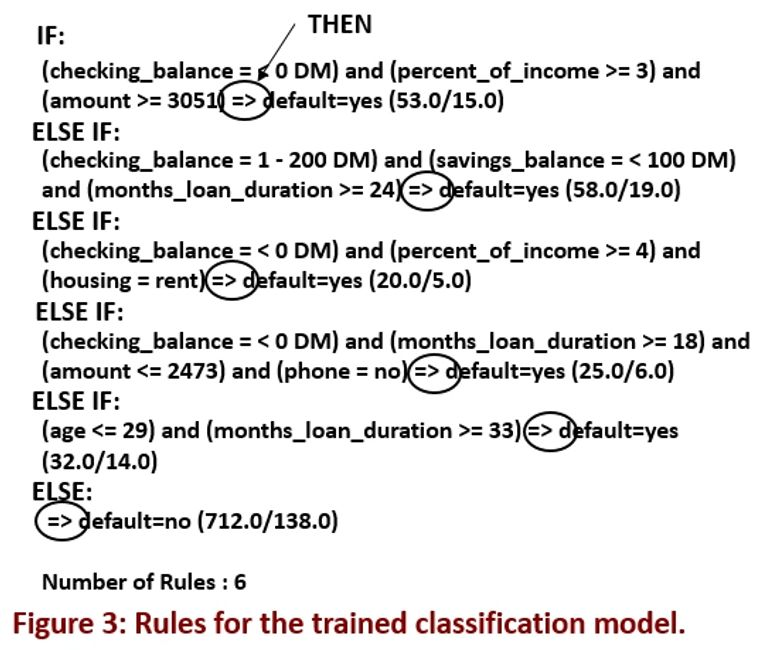There are six rules, whereas, the full decision tree unearthed 17 rules. To read the output we begin with the first rule. It would be read as IF-THEN. If the IF is not true, we pass to the second rule (ELSE-IF-THEN). Notice at each rule we can see the accuracy of the rule. For example, the first rule is a correct classification 71.6 percent ((53-15)/53) of the time.

There is a functional opportunity in the shorter list of rules as compared to decision tree models. The short is list is conducive to being placed along a process as a visual aid to assure correct actions of process operatives.

Just as for a decision tree model, we would evaluate the trained model against the holdout set of cases. A confusion matrix such at that previously shown in Figure 2 will come into play.

Now that we understand the two models, let’s dwell on the nature of actionable insight mined from misclassification in operational processes. The bank loan case is a good example of the degree of misclassification that can occur when there are dynamics that cannot be reflected as variables. The dynamics are the personal events of its borrowers.

The point is that the lending model reflects a much greater degree of misclassification then we would expect, thus, allow from procedural processes. Instead, a procedural process should reveal minimal misclassification. That is if the process is well designed and being well executed. If not, as revealed by the magnitude of misclassifications, there are questions to be asked and answered.

Why the revealed busts from the rules? Is it being revealed that the process does not actually deal with all of the realities of the work stream? Is it being revealed that we need to expand the classifications to capture the true comprehensive set of rules? Which rules, if broken along the process, will be least and most felt in the highest measures of profitability? The list of questions will be formed based on the familiarity of operatives, managers and SMEs with the subject process.

A final comment on methods. Neural network models can function as classification models. Any given model will not necessarily be any more accurate than a decision tree model or rule classification model. Even when more accurate, there is a deal-killer. We cannot know how it arrived at its classifications. It is essentially an unhelpful black box to us.

Underlying Subgroups to Process Measurements

The previous two methods to search for apparency worked backward from classifications to find the rules leading to each classification and its probability. In contrast, we can seek to make groups apparent within an outcome measurement and the rules that define them. What we are really doing is unearthing subgroups, each defined by a rule-measurement set.

Regression trees models” and “model trees” make it possible. Both present numeric measurements at each leaf. Although confusing in name, the leaves of a “regression” tree model report the averages of the observed cases. In contrast, a “model” tree produces a regression model at each leaf.

Figure 4 is a trained regression tree model for scoring how a wine would be rated by tasters as a relationship to the composite chemical elements found in all wines. At its leaves, we see the score for different compositions. Just as for decisions trees, the chemical compositions are the rules leading to the actual scores. We can imagine that sales volumes and prices could just as easily have been made the targeted measurement variable.

We read the tree just as for the decision tree model. However, the outcome is the average of all actual scores at each leaf. Also at the leaf is the number of observations. The percentage is the number of cases at each leaf of the total cases.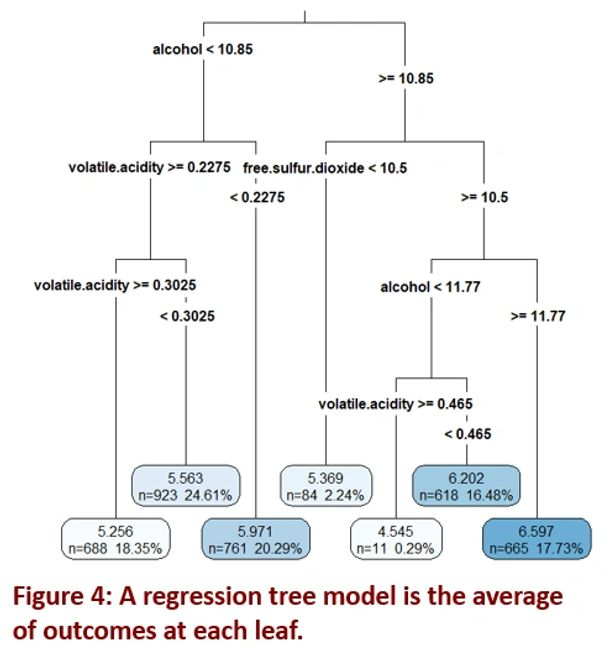Rather than get lost in the elegance of the tool, let’s focus on what it is we have in front of us. From the body of wines, we have identified subgroups of rules and outcomes.

Imagine the concept if it were applied to group work orders or other aspects of a process. Our targeted measurement variable could be hours, dollars, productivity, etc. Imagine that we want to explore the range of orders as to make up, type, etc. Imagine what you would do with such information in the design, resourcing and controlling an operational process.

Rather than an average of the history such as hours, costs, volumes, etc., each leaf presents as a regression model. This is done with a model tree as shown in Figure 5—truncated to graphically fit the confines of this article.

At each leaf, we are given a local linear regression model as shown as an insert to the figure. This allows us to explore the relative strength of all chemical elements as indicative variables in the model to predicting the score at the leaf.

Notice that the predicted regression includes all variables although the rules leading to the leaf engage fewer variables: five in the selected leaf. The team can subset the associated 266 observations from the dataset and build a local model now that the learned rules have identified the most important variables.The percentage at the leaf is a measure of accuracy at the branch called the “root relative squared error.” It is the sum of the absolute differences between actual and predicted cases, divided by the sum of the absolute differences of each actual case and the mean of all actual cases.

Just as for classifiers, models are evaluated for their ability to predict—assuming that prediction is to be the role of the model. A model is trained and then tested against a holdout set. Figure 3 introduced and explored the confusion matrix to evaluate decision trees and classification rule models. The matrix is, of course, nonsensical to the evaluation of regression trees and model trees.

Instead, the models are evaluated for how far their predictions are from the actual value. The measure is called “mean absolute error” (MAE). The sum of the differences between predicted and actual, as absolutes, is divided by the number of total observations in the model. The MAE for the full regression tree and the model tree are 0.58 and 0.54 respectively.

Before moving on, let’s set a stake in the ground of modeling. The example demonstrated a case in which all of the indicator variables are numeric. However, just as for decision trees, the indicator variables can include non-numeric categorical variables. Building models with mixtures of numeric and categorical variables is equally true for just about every type of model across the five questions; including the method to be explained next.

Underlying Variables to Captured Variables

Now there is another apparency question to be asked. Are there important variables to the operational process that lurk about beneath the surface and our ability to spot them? If we could bring them to the surface, we could apply them to the apparency questioning models of this article, as well as, the other four core types of questioning models for exploring operational relationships, differences, time series, and durations.

But how are we to find variables if they do not actually exist within the datasets we can pull from any of the firm’s standard operating systems? The answer lies in searching for variables which can be constructed from other variables in the system. The variables are called “clusters.”

The concept of the method is simple. It is most often determined with what is called a “K-Means model.” The “K” refers to the number of clusters we ask it to tease out of the training dataset we give it. There are many variations of the analytic, but K-means is the most mainstream and will serve just about every exploration in operational excellence. Let’s also make note that text mining is possible with the K-means model.

Figure 6 shows the case of a survey of employee approval ratings for six factors and overall. To make the method clear to us, the case of only two ratings (learning and advancement) is shown in Figure 6. The lines demarcate the clusters as learned by the K-means model.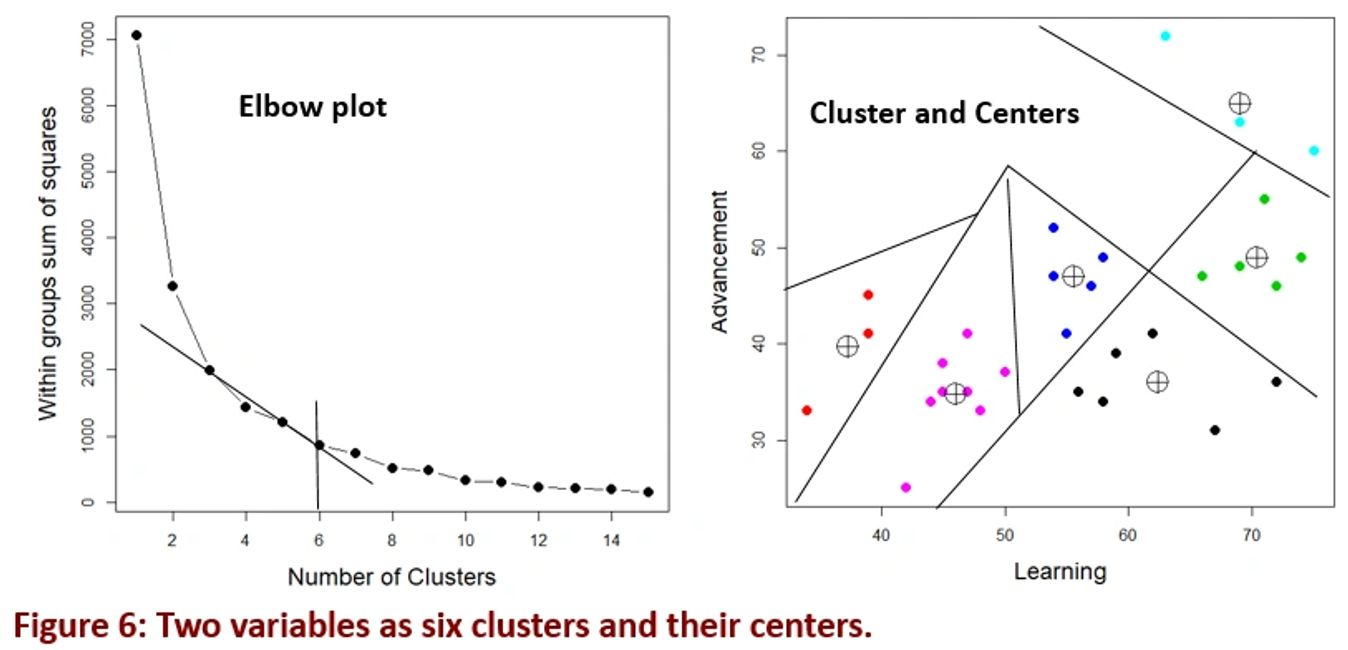The model learns the best fitting centers among clusters. However, before that we need an analytic to decide how many clusters (K) to ask the K-means model to tease out of the two variables. In other words, beyond how many clusters is there no truly significant gained incremental insight?

The left panel of the figure shows what is called the “elbow” plot. Each point is a K. We look for the deflection point at which an additional K provides little additional value. In this case, the plot suggests that we seek six clusters (K=6) as shown at the break from the tangent line.

The right panel shows the six clusters that the K-Means model has learned that exist hidden in the dataset. Each has a center as shown. Around each center, as a cluster, are the mathematically closest cases to the center.

Because cluster models are unsupervised, now the team must use its expertise and knowledge of the process to give the clusters a meaning. In this case, the meaning would somehow reflect how the two variables are related with respect to each cluster.

The clusters are a new classification variable to us. Alternatively, they can be played as a new indicator variable to a known target variable.

Now let’s extend the concept beyond the two dimensions (variables). We can seek clusters across as many variables as we wish. Figure 7 shows the case of clusters on three variables. The points at each cluster are the intersection factors (centers) of three dimensions (x, y, z).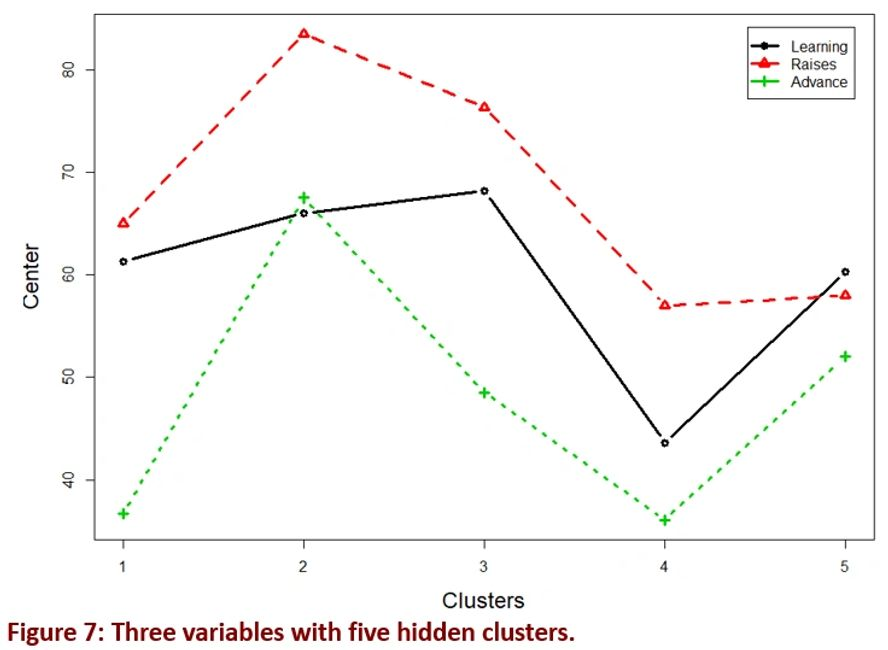The figure came about because, upon inspection of the seven variables, there seems to be aspects of satisfaction and dissatisfaction. We picked the three ratings associated with satisfaction—learning, raises and advancement.

Of course, it is increasingly difficult to visually interpret the clusters as the number of involved variables increases. The figure shows only one of many creative ways to visually explore the clusters. Ideas for visualization are outside the scope of this article.

Although not shown, the associated elbow plot advised seeking five clusters. Consequently, the five clusters show different patterns at their respective three dimensional centers. The difference will send the team to explore why the clusters emerged and their meaning. In turn, the quest for meaning may send the team back to bring other variables into a clustering cycle.

Once joined with the master dataset, a powerful way to look for meaning in the clusters is to apply them as a target variable to the classification models introduced in this article. Another powerful strategy is to include the cluster variable as one of the variables for consideration as an important indicator variable to the range of models.

As already mentioned, the clusters are actually a new variable to be joined with the source dataset. This opens the way to deeper exploration. However, most important is that these explorations take place below the surface as the variable is pulled into all other types of questioning and their enabling models—relationship, difference, time, and duration along with apparency.

Finally

What we have is a real boon; one we have never had before. Apparency questions unearth what we did not know to consider in the design, workings and control of operational processes. This results in a much more target-rich universe of opportunities to advance business success. Furthermore, the new targets we introduce for consideration will be bump down the less attractive opportunities that were before ranked higher on a master list of opportunities.

Sources for self-directed learning: Discovering Statistics Using R, Field and Miles, 2012 | Multilevel Modeling Using R, Holmes, 2014 | Machine Learning with R, Lantz, 2015 | ggplot2, Elegant Graphics for Data Analysis, Wickham, 2016 | Introductory Time Series with R, Cowpertwait and Metcalfe, 2009 | Event History Analytics with R, Bostrom, 2012 | Package “tsoutliers,” Javier López-de-Lacalle, 2017